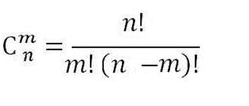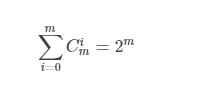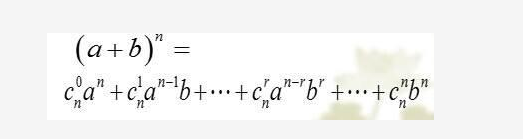• 组合数性质的证明Ⅰ

千次阅读 2017-10-06 20:19:02
若没有阅读上一章的内容请点这里 先列出来展开式 A(n,m)=n×(n-1)×…×(n-m+1)=n!/(n-m)!　① C(n,m)=A(n,m)/m!...组合数性质证明Ⅰ 　 ⑴对于0≤m≤n,有C(n,m)=C(n,n-m) 这是显而易见

若没有阅读上一章的内容请点这里

先列出来展开式

C(n,m)=A(n,m)/m!=n!/[(n-m)!×m!]　　　　　　　　　　　　②

由于时间原因　在这一篇不会全证明完

+

=左边

这个式子所描述的意义就是，在母本忠有n个不同元素，要先在其中取出l个，再在l个中取出r个样本的取法的数量；但是wulala爱创新，所以它先从n个不同元素中先取出r个，再在剩下的n-r个元素中取出l-r个元素。其实这两种取法的结果，都是得到三组，分别为n-l个，l-r个和r个元素。二者的结果都是一样的，所以，无论中间如何取，结果都是一样的。依照乘法原理也就得出了结论

好的，这次的内容就到这里了，学生党时间不多，不能一次性全部打完，敬请谅解
展开全文组合数学
• 组合数性质

千次阅读 2016-07-21 16:58:28
华电北风吹 天津大学认知计算与应用重点实验室 2016-07-21一、组合数定义 从n个不同元素中取出m个元素的个数，用CmnC_n^m表示。...}二、组合数性质 Cmn=Cn−mnC_n^m=C_n^{n-m} Cmn=Cmn−1+Cm−1n−1C_n^m

华电北风吹
天津大学认知计算与应用重点实验室
2016-07-21

一、组合数定义
从n个不同元素中取出m个元素的个数，用 Cmn $C_n^m$表示。
Cmn=AmnAmm=n!m!(nm)! $C_n^m=\frac{A_n^m}{A_m^m}=\frac{n!}{m!(n-m)!}$

二、组合数性质
Cmn=Cnmn(1) $C_n^m=C_n^{n-m} \tag{1}$
Cmn=Cmn1+Cm1n1(2) $C_n^m=C_{n-1}^{m}+C_{n-1}^{m-1} \tag{2}$
mnCmn=Cm1n1(3) $\frac{m}{n}C_n^m=C_{n-1}^{m-1} \tag{3}$

三、练习
Cmm+Cmm+1+Cmm+2+Cmm+3+...+Cmn=Cm+1n+1(2)() $C_{m}^m+C_{m+1}^m+C_{m+2}^m+C_{m+3}^m+...+C_{n}^m=C_{n+1}^{m+1}(应用性质2){\tag{}}$

四、实例
从1到n中，随机挑选m个数，其中最大的那个数的期望是多少？
nk=mkCm1k1Cmn=m(n+1)m+1() $\frac{\sum_{k=m}^{n}{kC_{k-1}^{m-1}}}{C_n^m}=\frac{m(n+1)}{m+1}\tag{}$

展开全文• 2021_2022学年新教材高中数学第三章排列组合与二项式定理3.1.3.1组合与组合数及组合数性质课件新人教B版选择性必修第二册202107062122
• 组合数的各种性质和定理

万次阅读 多人点赞 2017-07-23 19:21:30
组合数有很多很多的性质和定理。。。 注意由于本人沉迷玩梗无法自拔，如果看见您看不懂的梗请随意跳过。 组合数通项公式 Cnm=m!n!∗(m−n)!Cmn=m!n!∗(m−n)!C_m^n=\frac{m!}{n!*(m-n)!} 证明：现在我们从m个...

从m个物品里选出n个的方案数，记作 Cnm C m n $C_m^n$，即为组合数
组合数有很多很多的性质和定理。。。
注意由于本人沉迷玩梗无法自拔，如果看见您看不懂的梗请随意跳过。

组合数通项公式

Cnm=m!n!(mn)! C m n = m ! n ! ∗ ( m − n ) !

证明：现在我们从m个不同的数里选出n个数组成一个排列，第一个位子上的数有m种取法，第二个位子上的有m-1种，第三个位子上有m-2种。。。共有 m!(mn)! m ! ( m − n ) ! $\frac{m!}{(m-n)!}$
然而，我们对于顺序没有要求，假设取出了n个数，第一个位子上有n种放法，第二个位子上有n-1种。。。所以我们刚才得到的哪个东西还要除一个 n! n ! $n!$

组合数递推公式

Cnm=Cnm1+Cn1m1 C m n = C m − 1 n + C m − 1 n − 1

证明：从m个不同的数中取n个，第m个数如果取的话有 Cn1m1 C m − 1 n − 1 $C_{m-1}^{n-1}$种取法，如果不取则有 Cnm1 C m − 1 n $C_{m-1}^n$种。
如果您是组合数新手，请牢记以上两个公式

性质1

Cnm=Cmnm C m n = C m m − n

证明：显然从m个物品里选n个和从m个物品里选m-n个丢掉的方案数是一样的。

性质2

Crm+r+1=i=0rCim+i C m + r + 1 r = ∑ i = 0 r C m + i i

证明：用组合数的递推公式。
首先 C0m=C0m+1=1 C m 0 = C m + 1 0 = 1 $C_m^0=C_{m+1}^0=1$
C0m+C1m+1+C2m+2+...+Crm+r C m 0 + C m + 1 1 + C m + 2 2 + . . . + C m + r r $C_m^0+C_{m+1}^1+C_{m+2}^2+...+C_{m+r}^r$=
C1m+C1m+1+C2m+2+...+Crm+r C m 1 + C m + 1 1 + C m + 2 2 + . . . + C m + r r $C_m^1+C_{m+1}^1+C_{m+2}^2+...+C_{m+r}^r$=
C1m+2+C2m+2+...+Crm+r C m + 2 1 + C m + 2 2 + . . . + C m + r r $C_{m+2}^1+C_{m+2}^2+...+C_{m+r}^r$=
Crm+r+1 C m + r + 1 r $C_{m+r+1}^r$

性质3

CnmCrn=CrmCnrmr C m n ∗ C n r = C m r ∗ C m − r n − r

证明:用组合数的通项公式
CnmCrn= C m n ∗ C n r = $C_m^n*C_n^r=$
m!n!(mn)!n!r!(nr)!= m ! n ! ( m − n ) ! ∗ n ! r ! ( n − r ) ! = $\frac{m!}{n!(m-n)!}*\frac{n!}{r!(n-r)!}=$
m!r!(mr)!(mr)!(mn)!(nr)!= m ! r ! ( m − r ) ! ∗ ( m − r ) ! ( m − n ) ! ( n − r ) ! = $\frac{m!}{r!(m-r)!}*\frac{(m-r)!}{(m-n)!(n-r)!}=$
CrmCnrmr C m r ∗ C m − r n − r $C_m^r*C_{m-r}^{n-r}$

性质4（二项式定理）

i=0mCim=2m ∑ i = 0 m C m i = 2 m

证明：显然 Cim C m i $C_m^i$代表一个m位二进制数有i个0的情况下的数量，那么这个和就是m位二进制数的数量了。
推广一下这个二项式定理：
i=0mCimxi=(x+1)m ∑ i = 0 m C m i ∗ x i = ( x + 1 ) m

这个又怎么证明呢？先把 (x+1)m ( x + 1 ) m $(x+1)^m$写成 (x+1)(x+1)(x+1)... ( x + 1 ) ( x + 1 ) ( x + 1 ) . . . $(x+1)(x+1)(x+1)...$的格式，然后每一项很精妙啊，比如说i次方项，选的 i i $i$$x$$x$是从哪个括号里来呢？有 Cim C m i $C_m^i$种方案吧，所以 xi x i $x^i$项的系数是 Cim C m i $C_m^i$
这就是杨辉三角的应用（可以自行百度）

性质5

C0mC1m+C2m...±Cmm=0 C m 0 − C m 1 + C m 2 − . . . ± C m m = 0

证明：假如m是奇数的花，由性质1可知正确。
假如m是偶数的花，这个里面的花，用一下递推公式,然后显然 C0m1=C0m=1 C m − 1 0 = C m 0 = 1 $C_{m-1}^0=C_m^0=1$并且 Cm1m1=Cmm=1 C m − 1 m − 1 = C m m = 1 $C_{m-1}^{m-1}=C_m^m=1$，则：
C0mC1m+C2m...+Cmm= C m 0 − C m 1 + C m 2 − . . . + C m m = $C_m^0-C_m^1+C_m^2-...+C_m^m=$
C0m1C0m1C1m1+C1m1+C2m1...+Cm1m1=0 C m − 1 0 − C m − 1 0 − C m − 1 1 + C m − 1 1 + C m − 1 2 − . . . + C m − 1 m − 1 = 0 $C_{m-1}^0-C_{m-1}^0-C_{m-1}^1+C_{m-1}^1+C_{m-1}^2-...+C_{m-1}^{m-1}=0$

性质6

C0m+C2m+C4m...=C1m+C3m+C5m+...=2m1 C m 0 + C m 2 + C m 4 . . . = C m 1 + C m 3 + C m 5 + . . . = 2 m − 1

证明：这个根据性质4和性质5可知正确。

性质7

Crm+n=C0mCrn+C1mCr1n++CrmC0n C m + n r = C m 0 ∗ C n r + C m 1 ∗ C n r − 1 + … + C m r ∗ C n 0

证明：很简单，考虑我选出的r个物品在前m个物品有几个，在后n个物品里有几个即可。
特别的：
Cnm+n=C0mC0n+C1mC1n++CmmCmn C m + n n = C m 0 ∗ C n 0 + C m 1 ∗ C n 1 + … + C m m ∗ C n m

这个是根据性质1变形得到的。

性质8

nCnm=mCn1m1 n ∗ C m n = m ∗ C m − 1 n − 1

证明：运用通项公式
nCnm= n ∗ C m n = $n*C_m^n=$
nm!n!(mn)!= n ∗ m ! n ! ( m − n ) ! = $n*\frac{m!}{n!(m-n)!}=$
m!(n1)!(mn)!= m ! ( n − 1 ) ! ( m − n ) ! = $\frac{m!}{(n-1)!(m-n)!}=$
m(m1)!(n1)!(mn)!=mCn1m1 m ∗ ( m − 1 ) ! ( n − 1 ) ! ( m − n ) ! = m ∗ C m − 1 n − 1 $m*\frac{(m-1)!}{(n-1)!(m-n)!}=m*C_{m-1}^{n-1}$

性质9

i=1nCini=n2n1 ∑ i = 1 n C n i ∗ i = n ∗ 2 n − 1

证明：用通项公式
ni=1Cini=n2n1= ∑ i = 1 n C n i ∗ i = n ∗ 2 n − 1 = $\sum_{i=1}^n C_n^i*i=n*2^{n-1}=$
ni=1n!(i1)!(ni)!= ∑ i = 1 n n ! ( i − 1 ) ! ( n − i ) ! = $\sum_{i=1}^n \frac{n!}{(i-1)!(n-i)!}=$
(ni=1(n1)!(i1)!(ni)!)n= ( ∑ i = 1 n ( n − 1 ) ! ( i − 1 ) ! ( n − i ) ! ) ∗ n = $(\sum_{i=1}^n \frac{(n-1)!}{(i-1)!(n-i)!})*n=$
(n1i=0Cin)n= ( ∑ i = 0 n − 1 C n i ) ∗ n = $(\sum_{i=0}^{n-1} C_n^i)*n=$
现在看性质4。
n2n1 n ∗ 2 n − 1 $n*2^{n-1}$

性质10

i=1nCini2=n(n+1)2n2 ∑ i = 1 n C n i ∗ i 2 = n ∗ ( n + 1 ) ∗ 2 n − 2

证明
和上一个性质有些类似。
ni=1Cini2= ∑ i = 1 n C n i ∗ i 2 = $\sum_{i=1}^n C_n^i*i^2=$
用和上面差不多的方法得到：
(n1i=0Cin1(i+1))n= ( ∑ i = 0 n − 1 C n − 1 i ∗ ( i + 1 ) ) ∗ n = $(\sum_{i=0}^{n-1} C_{n-1}^i*(i+1))*n=$
(n1i=0Cin1i+n1i=0Cin1)n= ( ∑ i = 0 n − 1 C n − 1 i ∗ i + ∑ i = 0 n − 1 C n − 1 i ) ∗ n = $(\sum_{i=0}^{n-1} C_{n-1}^i*i+\sum_{i=0}^{n-1}C_{n-1}^i)*n=$
用性质9和性质4可以得到：
(2n2(n1)+2n1)n= ( 2 n − 2 ∗ ( n − 1 ) + 2 n − 1 ) ∗ n = $(2^{n-2}*(n-1)+2^{n-1})*n=$
很明显 2n1=22n2 2 n − 1 = 2 ∗ 2 n − 2 $2^{n-1}=2*2^{n-2}$
所以原式= 2n2(n+1)n 2 n − 2 ∗ ( n + 1 ) ∗ n $2^{n-2}*(n+1)*n$

性质11

i=0n(Cin)2=Cn2n ∑ i = 0 n ( C n i ) 2 = C 2 n n

证明：boshi说，他的门前有两棵树， 一棵是枣树，另一棵也是枣树，每棵树上有n个枣子，每个枣子都有一个不同的神奇的膜法符号。现在boshi从两棵树上一共打下了n个枣子，那么一共有多少种方案？是 Cn2n C 2 n n $C_{2n}^n$,也是第一棵树上打下i个枣子，从第二棵树上打下（n-i）棵枣子的方案，根据乘法原理乘起来，又因为 Cin=Cnin C n i = C n n − i $C_n^i=C_n^{n-i}$，所以得到上一个式子。

卢卡斯定理

简单的说就是求 Cnm%p C m n % p $C_m^n \% p$的时候可以化作 Cnm=Cn/pm/pCn%pm%p C m n = C m / p n / p ∗ C m % p n % p $C_m^n =C_{m/p}^{n/p} *C_{m \% p}^{n \% p}$，那么只要递归 Cn/pm/p C m / p n / p $C_{m/p}^{n/p}$即可。
证明我蠢我不会自己想

后记

啊啊啊搞了一下午终于证完了累死了。。。感觉自己和组合数的感情有了明显的提升（才怪）。。。
在文章的最后%一发数王。。。
%%%%%%%%%数王您太强了%%%%%%%%%%%
数王说以上所有定理都可以用那个那个那个证，虽然我不知道那个是哪个，但是反正好强啊%%%%%%%%
好吧以上都是不正经内容，正经内容是：
在做题的时候大家可能不一定都会遇到这些性质，但是在手动证明完这些性质后对于组合数变形的问题就会有更深一层的理解，总之，组合数性质可以用一下方法推出：
1.情景假设法（假设boshi从枣树选枣子的方案。。。
2.隔板法（boshi把枣子放成一排，通过在枣子间添加隔板来分组。。。
3.通向公式法
4.递推公式法
以上。

展开全文组合数学
• 首先我们都知道组合数的意义，就是说一共有n个样本，一次性从中取出m个样本，一共有多少种不同的取法。它的公式如下： 它有这么一个性质： 该性质有若干种证明方式，今天我在这边写出我觉得挺巧妙的一种证明方式。...

1.公式

• 首先我们都知道组合数的意义，就是说一共有n个样本，一次性从中取出m个样本，一共有多少种不同的取法。它的公式如下：它有这么一个性质：该性质有若干种证明方式，今天我在这边写出我觉得挺巧妙的一种证明方式。

2.证明

• 想必大家都知道有关的另一个公式：• 关于这个公式的系数（也就是c(n,0),c(n,1)…）可以这么理解：
首先知道，(a+b) ^n 的展开式一共有n+1项，分别是a ^n，a ^n-1b，…ab ^n-1，b ^n。
(a+b) ^n 就是有n个（a+b）相乘，相当于(a+b)(a+b)(a+b)…(a+b)(a+b)，一共n个。
对于a^n，相当于是从n项中找n个a相乘，其系数就是C(n,0)=1，
对于a^n-1
b，相当于是从n项中找 (n-1) 个a和 1 个b相乘，其系数就是C(n,1)=n，
对于a^n-2*b ^2，相当于是从n项中找 (n-2) 个a和 2 个b相乘，其系数就是C(n,2)，

对于b^n，相当于是从n项中找 n 个b相乘，其系数就是C(n,n)=1，

• 由此可知：组合数之和 = 二项式的系数之和 = （a+b）^n一共的项数
很明显，（a+b）^n，一共有2 ^n个系数为1的项

（a+b）^n
n=12项
n=24项
n=38项
n=k2^k项

这也算是加深了自己的理解吧，以前一直只知道死记公式，从不想为什么，今后这毛病得改。。。

展开全文组合数性质 二项式定理证明
• 组合数及其性质和证明

千次阅读 2019-06-26 15:56:55
组合数 从 nnn 个不同元素中，任取 m(m≤n)m(m≤n)m(m≤n) 个元素并成一组，叫做从 nnn 个不同元素中取出 mmm 个元素的一个组合；从 nnn 个不同元素中取出 m(m≤n)m(m≤n)m(m≤n) 个元素的所有组合的个数，叫做从 ...
• 组合数的性质&证明

千次阅读 2017-08-06 18:15:46
• 组合数公式的详细推导

千次阅读 2020-04-13 17:51:32
组合数： （详细百度科普点我） 从n个不同元素中，任取m(m≤n)个元素并成一组，叫做从n个不同元素中取出m个元素的一个组合;从n个不同元素中取出m(m≤n)个元素的所有组合的个数，叫做从n个不同元素中取出m个元素的...线性代数 c++
• 组合数的推广

千次阅读 2016-06-28 20:23:16
一直只以为组合数只是用于自然数集。后来发现这个世界无奇不有(简直变态)组合数CmnC_n^m下标n已经推广到实数集，m推广到整数集。 个人觉得有些部分的应用不是很广，但下标n，m在整数集的应用挺重要的，有必要了解...
•杨辉三角形
• 组合数算法

千次阅读 2017-08-22 18:22:49
组合数计算可以用过阶乘然后得出结果，但是由于计算机数据存储方式的限制，阶乘的计算，如果是long int型只能正确计算到12左右的阶乘，如果用double型只能正确计算170左右的阶乘，当然这些只是大概，需要结合实际...算法 组合数
• C++求解组合数的具体实现

千次阅读 多人点赞 2020-09-20 12:54:34
很少写关于具体算法的总结笔记，因为很难把一个算法...这次想总结一下组合数的具体实现，原因是最近总是碰见组合数，所以决定来写写，免得每次从头推导公式耽误时间。排列组合经常会作为一个问题解决方案中一部分...C++ 算法 组合数 递归 动态规划
• 计算组合数的三种方式

千次阅读 多人点赞 2020-03-19 02:19:52
本文将详细介绍计算组合数的三种方式，这三种方式分别适用于不同的场景。组合数 卢卡斯定理 乘法逆元 java 费马小定理
• 2020_2021学年新教材高中数学第三章排列组合与二项式定理3.1.3.1组合与组合数组合数性质课时素养检测含解析新人教B版选择性必修第二册20210329227
• Java计算组合数以及生成组合排列

万次阅读 多人点赞 2018-11-24 00:28:29
组合数计算 公式法 逐个相除法(错误) 逐个相除法修正版 素数幂乘法 基本公式法 平方差连乘法 组合恒等法 简单递归法 杨辉三角法 杨辉三角优化法 二进制法 组合数计算小结 获取数组的组合排列 二进制法...Java 算法
• 组合数原理

千次阅读 2018-08-02 12:09:05
组合数 从n个不同元素中，任取m(m≤n)个元素并成一组，叫做从n个不同元素中取出m个元素的一个组合；从n个不同元素中取出m(m≤n)个元素的所有组合的个数，叫做从n个不同元素中取出m个元素的组合数。 公 式：C(n,m)...组合数
• 常用组合数计算公式及推算

万次阅读 多人点赞 2020-04-27 13:43:14
组合数的通项公式： 公式1： 证明： n个不同的数选择m个，第m个的选择方案为： 1、选第m个： 2、不选第m个: 公式2： 证明： 性质3： 证明： 性质4： 证明： 性质5： ...
• 组合数取模总结

千次阅读 2018-12-07 08:49:16
中，经常会遇到组合数取模的题目；就我现在的水平而言，大概分为以下三类，以后遇到新的方法会在做补充； 第一种： n和m都较小 （&lt;1000）,在这个数据范围内，我们可以直接用杨辉三角O(n^2)的复杂度内预处理...
• 快速求组合数

千次阅读 2019-05-13 13:34:54
所以这里要介绍一个种新的求组合算法。在了解此算法之前，要先了解一些概念。 1 同余 同余是数论中的重要概念。 给定一个正整数m，如果两个整数a和b满足a-b能够被m整除，即(a-b)/m得到一个整数，那么就称整...
• 组合数与杨辉三角形（帕斯卡三角形）的关系，此文面向初学者，推导了下组合数的递推公式。。然后又讲了讲排列组合相关的知识杨辉三角形 组合数 LaTeX
• 求组合数的6种方法

千次阅读 2020-02-01 14:37:06c++ 算法
• 组合数 从n个不同元素中，任取m(m≤n)个元素并成一组，叫做从n个不同元素中取出m个元素的一个组合；从n个不同元素中取出m(m≤n)个元素的所有组合的个数，叫做从n个不同元素中取出m个元素的组合数。 公式：C(n,m)=n!...
• C++实现计算组合数（详细）

千次阅读 2020-04-17 21:37:38
题目： 计算两个非负整数n和m，返回组合数。例如当n < 25,m = 12 时，答案为5200300。 计算组合数的公式： 先从排列数说起，排列数指从n个不同的...组合数和排列数的区别在于组合数与元素的顺序无关，排列数...
• 组合数

千次阅读 2017-08-07 16:28:53
1、定义：从m个不同元素中，任取n(n≤m)个元素并成一组，叫做从m个不同元素中取出n个元素的一个组合；从m个不同元素中取出n(n≤m)个元素的所有组合的个数，叫做从m个不同...互补性质：组合数性质如上图所示： 即从m
• 组合数奇偶性判定方式

千次阅读 2017-09-03 14:17:41
组合数的奇偶性判定方法为:  公式P是指排列，从N个元素取R个进行排列(即排序)。 (P是旧用法，现在教材上多用A，Arrangement)   公式C是指组合，从N个元素取R个，不进行排列（即不排序）。 组合数的奇偶性判定...acm
• 几种组合数的常见处理方式

千次阅读 2020-06-02 18:15:36
前言： 组合数，大家初中就学过，...我们初中就学过，组合数有许多性质，也肯定知道组合数有一个递推公式，即 C(n,m)=C(n-1,m-1)+C(n-1,m)，简单解释一下，我们从 n 个物体里选出 m 个物体，那么对于 n 个物体中的其中
• 组合数公式（代码实现）

千次阅读 2020-01-23 16:34:26
比如求 CnmC_{n}^{m}Cnm​,代码如下： ll combi(int n,int m){//求组合数的函数 ll cnt = 1; for(int i = 0; i < m; i++){ cnt *= n - i; cnt /= i + 1; } return cnt; } ...算法
• java求排列组合数

万次阅读 2017-09-15 14:51:06
组合数性质 即从m个不同元素中取出n个元素的组合数=从m个不同元素中取出(m-n)个元素的组合数；即：运用互补性质可以简化组合数的计算量。 package com.lan.MathFunction; //求排列数组合数 public class Test { ...
• 想必大家都知道的组合数在正整数上有：    但很少有人知道这个公式在实数领域上也是成立的：  也就是说n！在实数上有定义  x！=(x+1)。。。。。(x)为伽马函数    下面问题转移到伽马函数上面了，但是...
• 组合数C(n,k)的求法总结 与组合数有关的两个最重要内容是杨辉三角和二项式定理。 杨辉三角前10行如下所示： 另一方面，将(a+b)^n展开，系数正好和杨辉三角一致。 一般有(a+b)^n=C(n,0)a^n+C(n,1)a^(n-1)b+.....卢卡斯定理...